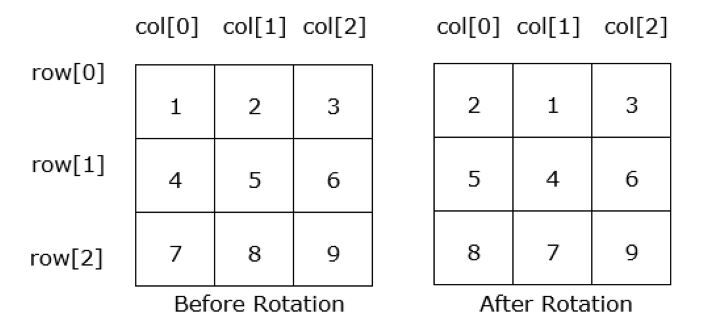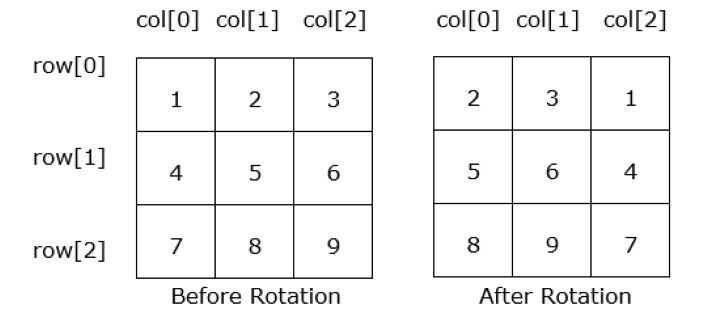# Java Program for Rotate the Matrix Right by K times

Array is a linear data structure that is used to store group of elements with similar datatypes. It stores data in a sequential manner. When we create an array of two dimensions, i.e., rows and columns, we call it matrix.

In this article, we will create a matrix of MxN and try to rotate it right by K times. Here, ‘M’ and ‘N’ are the size of the row and column respectively. ‘K’ is the number of times we want to rotate the matrix.

## Matrix Rotation to Right

Let’s understand what is matrix rotation with the following visual representation −

### Example 1

When we rotate the matrix 1 times −### Example 2

When we rotate the matrix 2 timesWe can see in the above diagrams that when we are rotating the matrix to right 1 time then, the first column gets shifted to 2nd position and when we are rotating the matrix to right 2 times then, the first column gets shifted to 3rd position.

### Syntax for Matrix

Data_Type matrix_name[ row ][ column ];
// declaration
Or,
// declaration with size
Data_Type matrix_name[ row ][ column ] = new Data_Type[sizeofarray];
Or,
// declaration and initialization
Data_Type matrix_name[ row ][ column ] = { {values separated by comma} };


We can use any of the above syntax in our program.

### Algorithm

Let’s discuss the approach how we are going to rotate a given matrix.

• Step 1 − We will create four integer values ‘row’ and ‘column’ of size 4. Third integer value as ‘K’ for number of rotations and fourth named ‘temp’ to store value temporarily.

• Step 2 − Declare and initialize a 2D array arr[][].

• Step 3 − Now, we will take a while loops that will run till ‘k % col’ times i.e. number of times the rotation has to be done. When we rotate the array greater than ‘col’ the rotation does not affect the original array.

• Step 4 − Inside while loop we will take two for loop, the first loop will run till the size of row and during each iteration it will store the value of last column of matrix to the ‘temp’ variable. The second for loop will take the remaining column value and shift it to right.

• Step 5 − After execution of the second for loop we will store the value of the ‘temp’ variable in the 1st column of matrix.

• Step 6 − When both of the for loops finish their execution then we will decrement the k by 1. After that we will check whether k is greater than 0 or not. If it is greater than 0 then, again the for loops will repeat their execution and work as discussed in steps 4 and 5.

In the next section, we will see the implemention for the above approach.

## Program for Rotate the Matrix Right by K times

### Example

public class Mat {
public static void main(String[] args) {
int row=4;
int col=4;
int arr[][] = {{7, 2, 1, 3}, {6, 1, 3, 7}, {4, 9, 8, 0}, {8, 0, 1, 2}};
int k = 3 % col; // Number of rotation
int temp;
System.out.println("The given matrix: ");
// loop to print matrix value
for (int i = 0; i < row; i++) {
for (int j = 0; j < col; j++) {
System.out.print(arr[i][j] + " ");
}
System.out.println();
}
// loop to perform matrix rotation
while(k > 0){
for( int i = 0; i < row; i++) {
temp = arr[i][col-1];
// storing last column value to temp
for(int j = col-1; j > 0; j--) {
// reverse loop
arr[i][j] = arr[i][j-1];
// shifting column value to right
}
arr[i] = temp;
// storing temp value to array again
}
k--; // decementing k
}
System.out.println("After rotating the given matrix " );
// loop to print new matrix value
for (int i = 0; i < row; i++) {
for (int j = 0; j < col; j++) {
System.out.print(arr[i][j] + " ");
}
System.out.println();
}
}
}


### Output

The given matrix:
7 2 1 3
6 1 3 7
4 9 8 0
8 0 1 2
After rotating the given matrix
2 1 3 7
1 3 7 6 9 8 0 4
0 1 2 8


## Conclusion

In this article, we have understood what is matrix and how we can declare and initialize it so that we can work with matrices. Also, we have discussed a java program to rotate a matrix k times.

Updated on: 02-May-2023

203 Views